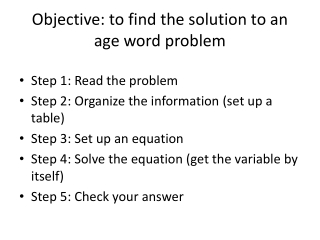DownloadDownload PresentationObjective: to find the solution to an age word problem

# Objective: to find the solution to an age word problem

Télécharger la présentation## Objective: to find the solution to an age word problem

- - - - - - - - - - - - - - - - - - - - - - - - - - - E N D - - - - - - - - - - - - - - - - - - - - - - - - - - -
##### Presentation Transcript

1. Objective: to find the solution to an age word problem • Step 1: Read the problem • Step 2: Organize the information (set up a table) • Step 3: Set up an equation • Step 4: Solve the equation (get the variable by itself) • Step 5: Check your answer

2. Age Word Problems A father is now 24 years older than his son. In 8 years, the father will be twice as old as his son will be then. Find their present ages. Equation: father = 2 son x+32 = 2(x+8)

3. Age Word Problems A father is 3 times as old as his son. Fifteen years ago, the father was 9 times as old as his son was then. Find their present ages. Equation: father = 9 son 3x-15 = 9(x-15)

4. Age Word Problems Phil is 24 years older than Stanley. Four years ago, he was 7 times as old as Stanley was then. Find their present ages. Equation: Phil = 7 Stanley x+20 = 7(x-4)

5. Age Word Problems Josephine is 22 years old and Ruth is 10 years old. In how many years will Josephine be twice as old as Ruth will be then? Equation: Josephine = 2 Ruth 22+x = 2(10+x)